Ex 9.3

Chapter 9 Class 12 Differential Equations
Serial order wise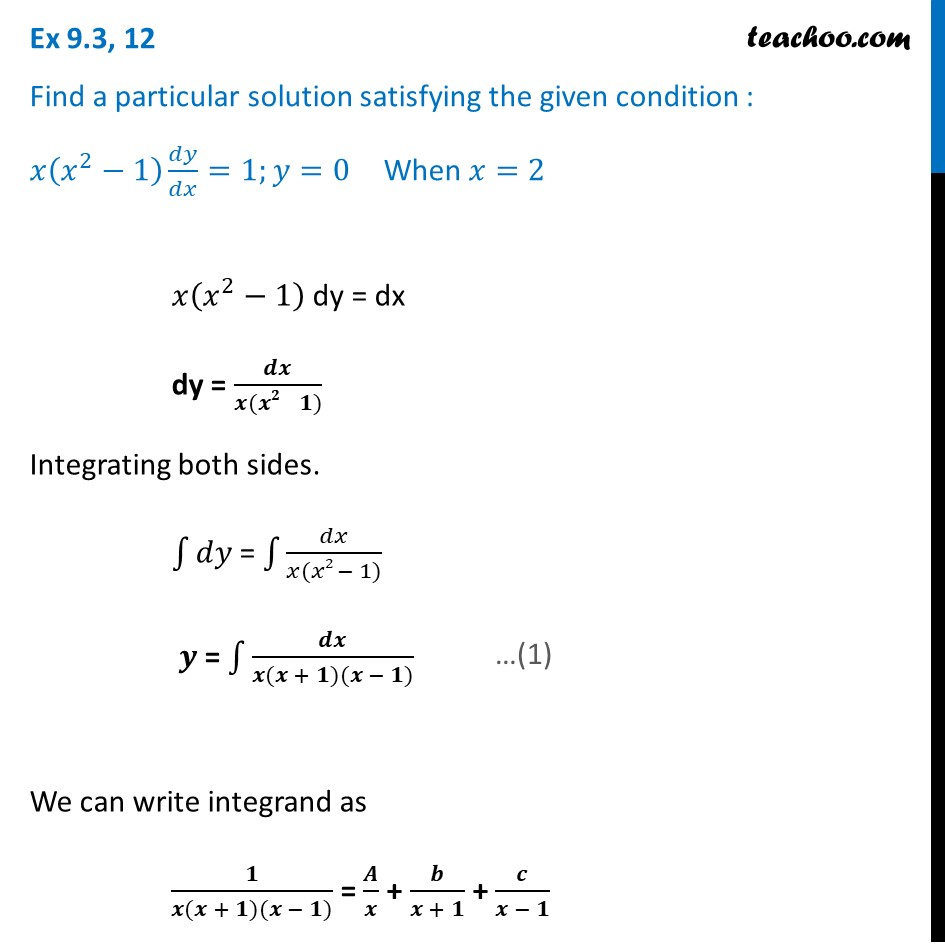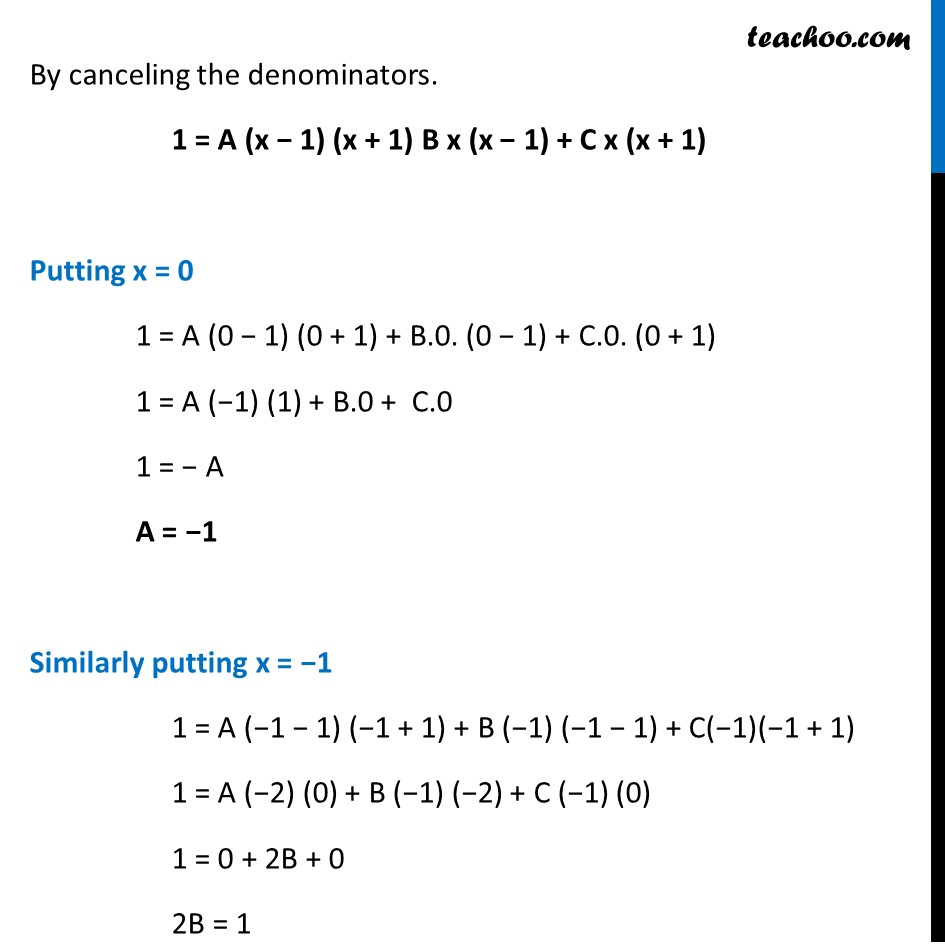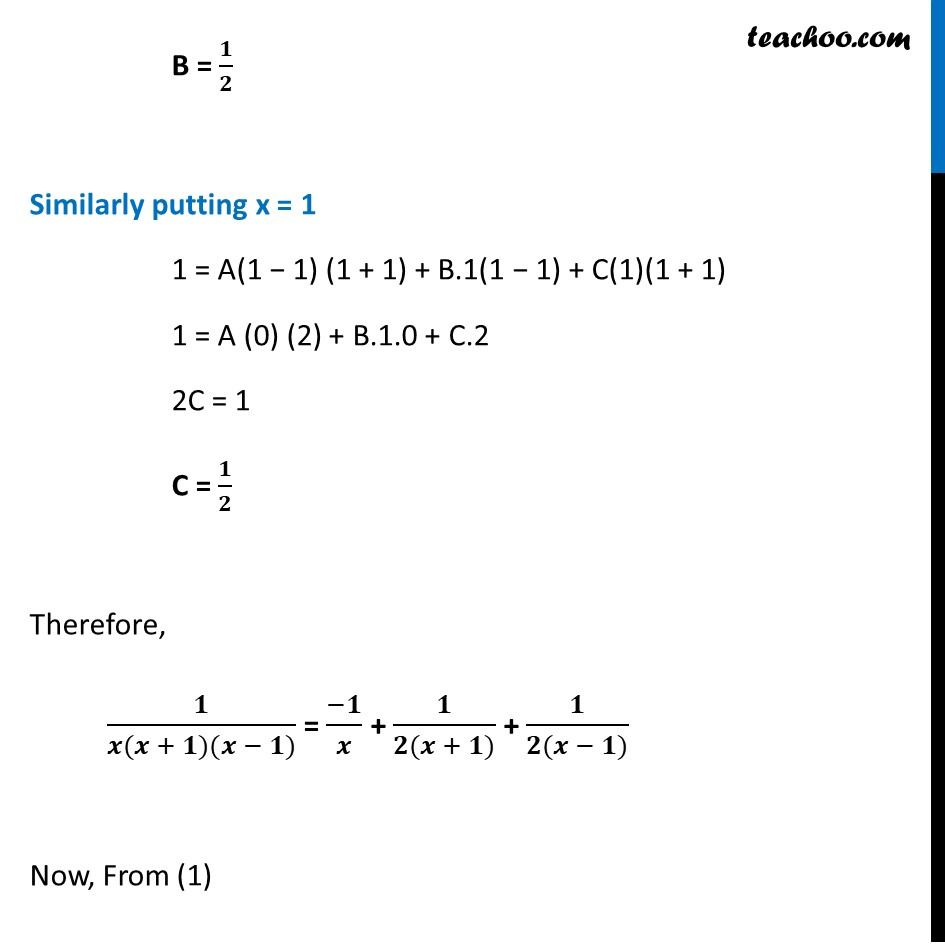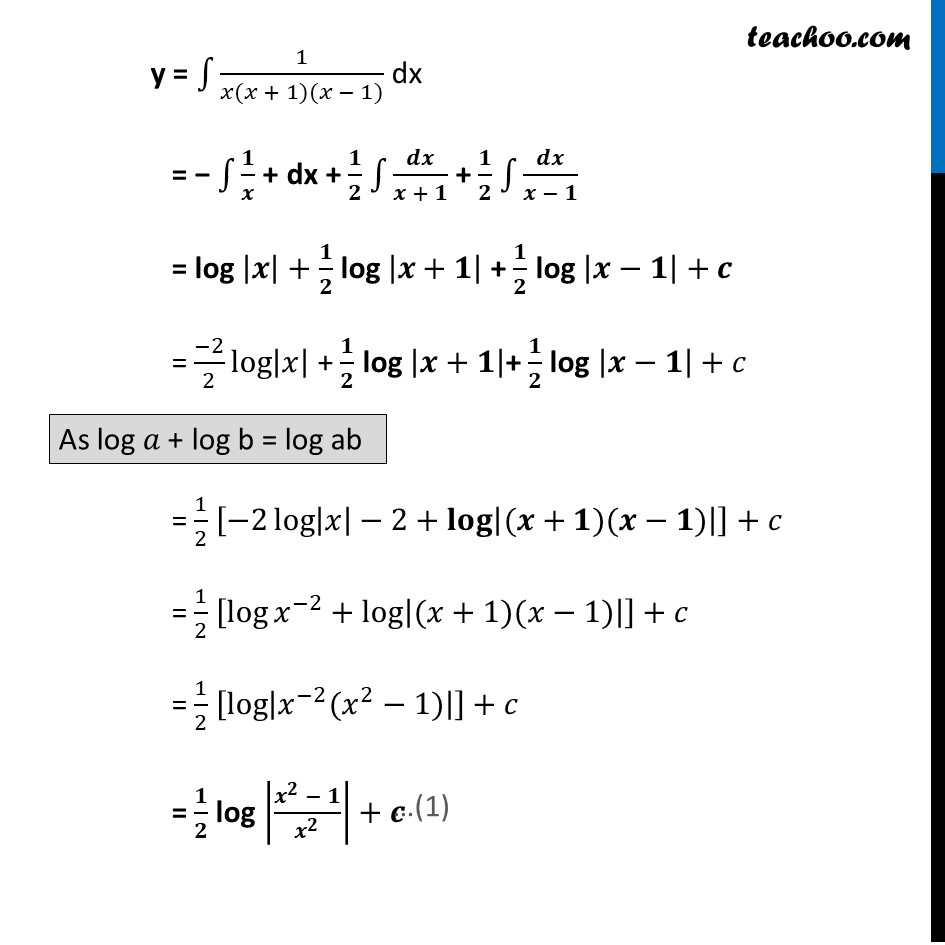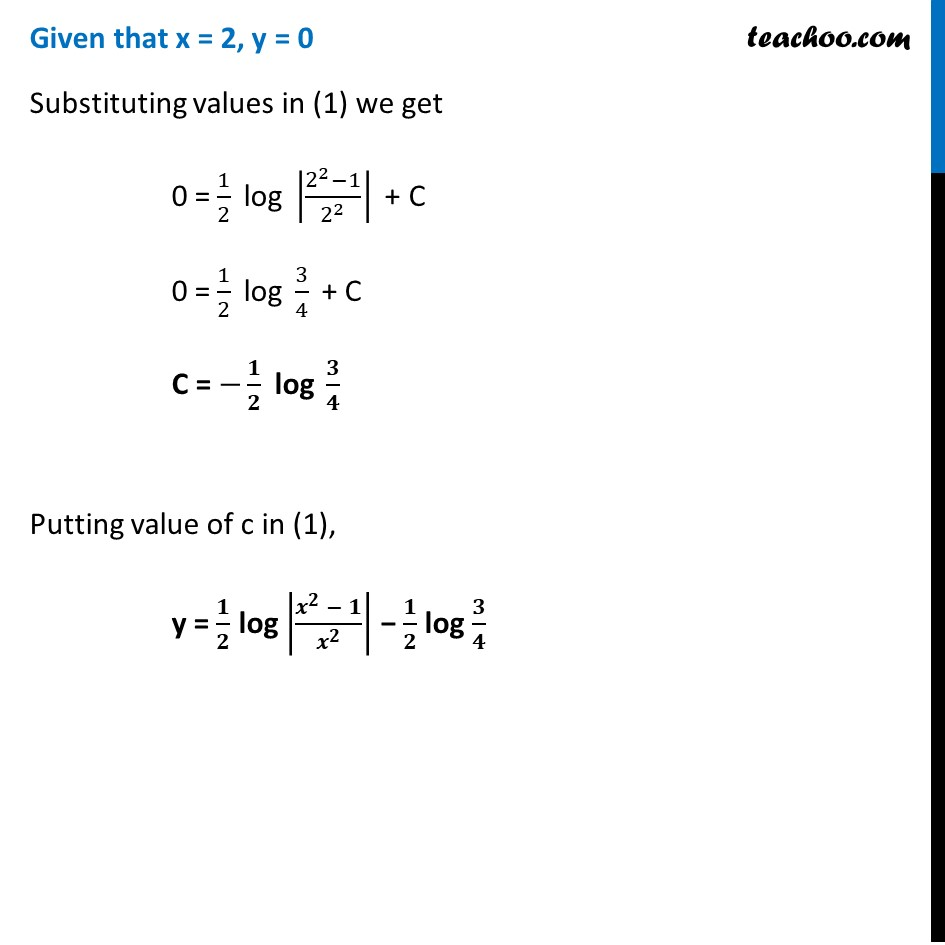Learn in your speed, with individual attention - Teachoo Maths 1-on-1 Class

### Transcript

Ex 9.3, 12 Find a particular solution satisfying the given condition : 𝑥(𝑥^2−1) 𝑑𝑦/𝑑𝑥=1;𝑦=0 When 𝑥=2 𝑥(𝑥^2−1) dy = dx dy = 𝒅𝒙/(𝒙(𝒙𝟐 − 𝟏)) Integrating both sides. ∫1▒𝑑𝑦 = ∫1▒𝑑𝑥/(𝑥(𝑥2 − 1)) 𝒚 = ∫1▒𝒅𝒙/(𝒙(𝒙 + 𝟏)(𝒙 − 𝟏)) We can write integrand as 𝟏/(𝒙(𝒙 + 𝟏)(𝒙 − 𝟏)) = 𝑨/𝒙 + 𝒃/(𝒙 + 𝟏) + 𝒄/(𝒙 − 𝟏) By canceling the denominators. 1 = A (x − 1) (x + 1) B x (x − 1) + C x (x + 1) Putting x = 0 1 = A (0 − 1) (0 + 1) + B.0. (0 − 1) + C.0. (0 + 1) 1 = A (−1) (1) + B.0 + C.0 1 = − A A = −1 Similarly putting x = −1 1 = A (−1 − 1) (−1 + 1) + B (−1) (−1 − 1) + C(−1)(−1 + 1) 1 = A (−2) (0) + B (−1) (−2) + C (−1) (0) 1 = 0 + 2B + 0 2B = 1 B = 𝟏/𝟐 Similarly putting x = 1 1 = A(1 − 1) (1 + 1) + B.1(1 − 1) + C(1)(1 + 1) 1 = A (0) (2) + B.1.0 + C.2 2C = 1 C = 𝟏/𝟐 Therefore, 𝟏/(𝒙(𝒙 + 𝟏)(𝒙 − 𝟏)) = (−𝟏)/𝒙 + 𝟏/(𝟐(𝒙 + 𝟏)) + 𝟏/(𝟐(𝒙 − 𝟏)) Now, From (1) y = ∫1▒1/(𝑥(𝑥 + 1)(𝑥 − 1)) dx = − ∫1▒𝟏/𝒙 + dx + 𝟏/𝟐 ∫1▒𝒅𝒙/(𝒙 + 𝟏) + 𝟏/𝟐 ∫1▒𝒅𝒙/(𝒙 − 𝟏) = log |𝒙|+ 𝟏/𝟐 log |𝒙+𝟏| + 𝟏/𝟐 log |𝒙−𝟏|+𝒄 = (−2)/2 log⁡|𝑥| + 𝟏/𝟐 log |𝒙+𝟏|+ 𝟏/𝟐 log |𝒙−𝟏|+𝑐 = 1/2 [−2 log⁡〖|𝑥|−2+𝐥𝐨𝐠⁡|(𝒙+𝟏)(𝒙−𝟏)| 〗 ]+𝑐 = 1/2 [log⁡〖𝑥^(−2)+log⁡|(𝑥+1)(𝑥−1)| 〗 ]+𝑐 = 1/2 [log⁡|𝑥^(−2) (𝑥^2−1)| ]+𝑐 = 𝟏/𝟐 log |(𝒙^𝟐 − 𝟏)/𝒙^𝟐 |+𝒄 Given that x = 2, y = 0 Substituting values in (1) we get 0 = 1/2 " log " |(2^2−1)/2^2 |" + C" 0 = 1/2 " log " 3/4 " + C" C = −𝟏/𝟐 " log " 𝟑/𝟒 Putting value of c in (1), y = 𝟏/𝟐 log |(𝒙^𝟐 − 𝟏)/𝒙^𝟐 | − 𝟏/𝟐 log 𝟑/𝟒